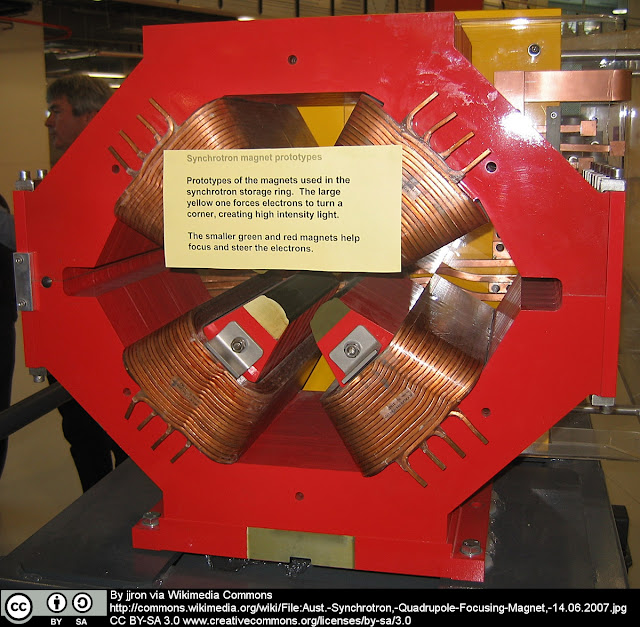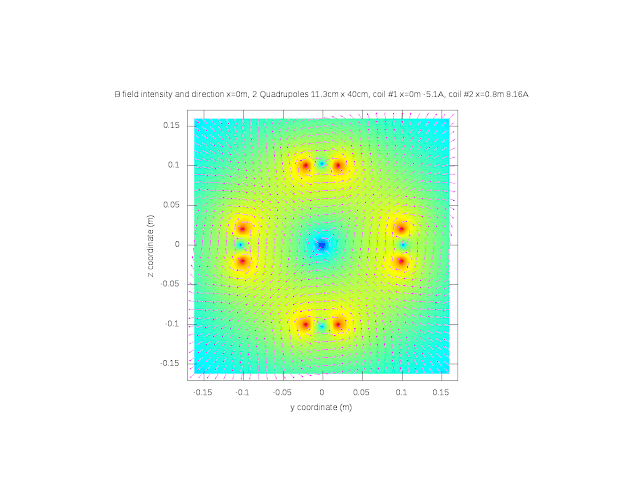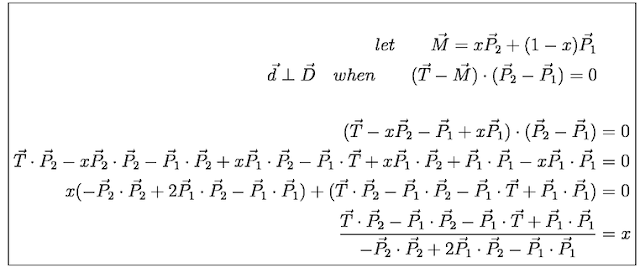## Thursday, May 24, 2012

### I hope you find this blog enjoyable and helpful. Support it with a small donation.

Over the last month I've done a series of posts about magnetic lenses and their ability to steer and focus electron beams.  The simulations are done in Octave and investigate electrons passing axially though single or multiple loops and how current can effect their trajectories.

Electron Beam Magnetic Lens Simulation
High Current Magnetic Lens Simulation
Multi-Turn Current Coil Magnetic Lens

While doing research I came across the following image of a quadrupole lens similar to the one used in the Australian Synchrotron.Quadrupole Lens

I was intrigued and thought I would try to simulate how the lens worked.  A quadrupole lens is conceptually basic, it consists of 4 multi-turn coils mounted on a high permeability core to direct and contain the magnetic field.  There are two north poles and two south poles, with opposing poles being of the same type.  The geometry for my simulation is going to be simpler.  I'll replace the multi-turn coils with single turn rectangular coils and leave out the core.  A cross section of the magnetic field produced is shown below.Quadrupole field cross section

This kind of lens can only focus one plane at a time, but as it does it will defocus the plane perpendicular to it.  For example, it will focus the beam in the horizontal direction, but defocus it in the vertical direction.  However, by using two quadrupoles with sufficient separation, one focussing vertically, and one focussing horizontally, the beam can be brought to a focus.

The code for my simulation isn't very pretty but it gets the job done.  In fact it is rather inefficient.  There are many opportunities to vectorise the code, but the 10 hours it took to run while I was at work cost me no time, whereas improving the code would have taken a couple of hours.  The simulation was split into separate parts, with data from the first stage saved to disk before continuing to the next stage.  The files can be found here.

To start off I have created a function called "B_Wire" that calculates the magnetic field created by a straight finite wire.  It takes three position vectors and a scalar as inputs.  The vector P1 is the start of the wire, P2 is the end of the wire, and T is the position where the magnetic field needs to be calculated.  I is the current in the wire.  With some vector operations, BT, the magnitude of the magnetic field can be easily calculated.Magnetic field magnitude calculation

The direction of the magnetic field can be calculated by first finding the position vector M.  The location of M is set by making vectors d and D perpendicular.  Once M is found, a vector in the direction of the magnetic field can be found by taking the cross product of (P2-M) and d.  A unit vector in the direction of the magnetic field can be found by normalizing the result.Calculating the position of M

However, as I write this I realise that I didn't need to do any of that.  By taking the cross product of the vectors C and D you get a vector in the direction of the magnetic field.  Oh well, either way should work.

The function BLens calculates the field of a single quadrupole via multiple calls to B_Wire.  The function B2Lens then calculates the field from 2 separate quadrupoles.  To examine the effects the quadrupole lenses have on an electron beam, 24 different trajectories with the same initial velocities in the x direction, and varying y z starting coordinates, were calculated.  By taking cross sections of the beams along the x axis the focussing effects can be observed.  The current in the second lens is in the opposite direction of the current in the first lens.  The magnitude of the current was found by trial and error.  Actual specifics aren't that important in this simulation as this is just to prove the concept.  The speed of electrons in a synchrotron is close to the speed of light, whereas in my simulation the electrons have a velocity of about 20% of that.  Combining that with the fact I have a simplified geometry, we can't take the numbers too seriously.

It can be seen that after the electrons pass through the first lens the beams start to focus in the z direction but defocus in the y direction.  As the beams pass through the next lens that focusses perpendicularly to the first one, the spreading of the beam in the y direction stops and the beams start to converge.  Convergence of the beams in the z direction imparted by the first lens starts to decrease, but isn't completely stopped.  The motion in the y and z direction is now balanced to bring the beam to a focus approximately 2.2 meters after the first lens.

Although the simulation uses a very simple model and does not accurately replicate how the lenses in a synchrotron work, the basic principles of operation can be seen.  The focussing of the beam in one plane and the defocussing of the beam in the plane perpendicular to it was observed.  Bringing the beam to a focus by using two quadrupole lenses was also demonstrated.

### Support me and the blog with a small donation. I appreciate each one.

#### 1 comment:

1.thank u blogger